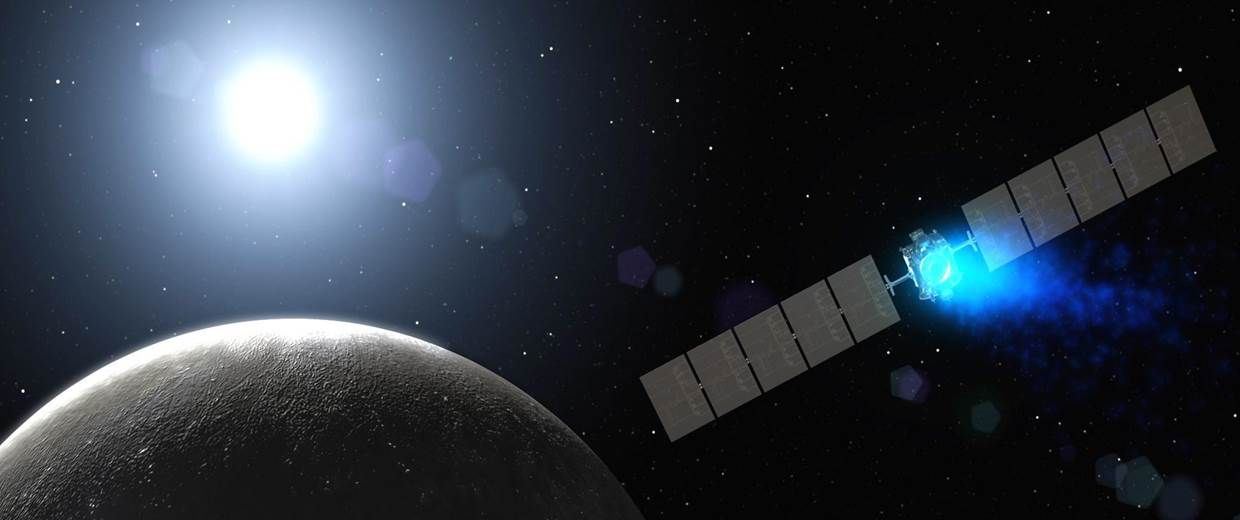# Making a Left Turn

An interplanetary probe speeding in deep space suddenly needs to make a left turn.It is presently traveling at $v= \SI[per-mode=symbol]{20000}{\meter\per\second}$, and needs to make a quarter circle turn, continuing at the same tangential speed. It is equipped with an ion thruster rocket capable of ejecting ions at $V= \SI[per-mode=symbol]{50000}{\meter\per\second}$. Moreover, it is a very advanced model that gets its ions from outer space so that the weight of the probe remains unchanged during its turn.

It takes a certain amount of total energy $E$ to propel the probe around this quarter circle turn. If the kinetic energy of the probe is $T=\frac{1}{2}m{v}^{2}$ (which remains unchanged throughout), what is the ratio $\dfrac{E}{T}?$ Submit your answer to 3 decimal places.


Details and Assumptions:

• Assume that no energy is gained or lost in the probe's acquisition of interplanetary ions before they are ejected by the ion thruster engine.
• Assume that the probe undergoes rotation during the turn so that the ion thrust always points outward from the center of the turn, again with no energy expended for this rotation.
• Assume that there are no gravitational masses nearby.
×

Problem Loading...

Note Loading...

Set Loading...